# Area of Parallelogram

Area of Parallelogram
Go back to  'Area'

A parallelogram is a special kind of quadrilateral.

Rectangle, square, and rhombus are parallelogram examples.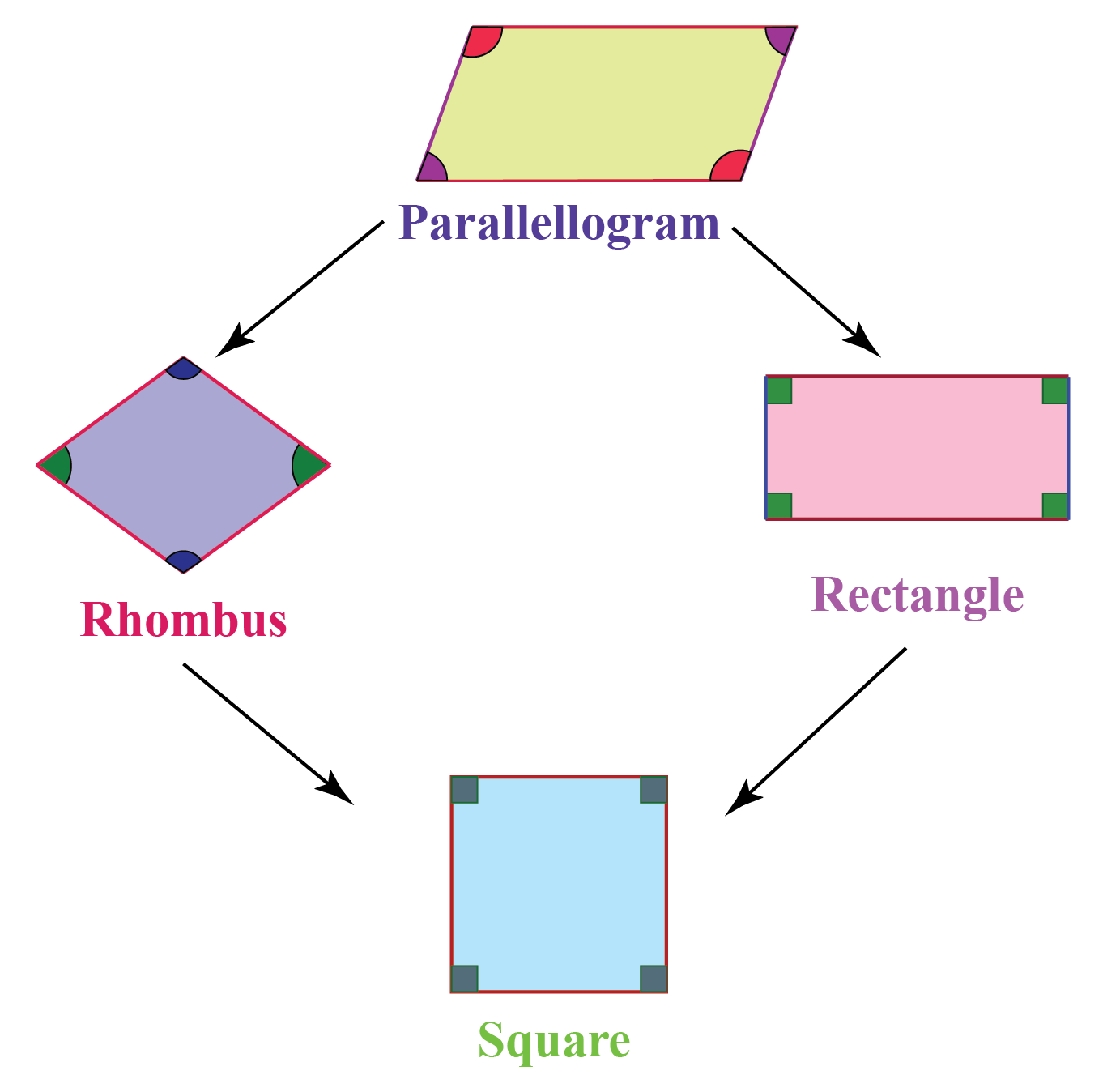If a quadrilateral has a pair of parallel opposite sides, then it is called as a Parallelogram.

Geometry is all about shapes, 2D or 3D. All of these shapes have a different set of properties with different formulas for area and perimeter.

The prime focus of this chapter will be entirely on the following:

• Definition of the area of a parallelogram
• Formula of the area of a parallelogram
• Real-world examples related to the area of a parallelogram

Once you get these concepts, you will then learn about the area of parallelogram using vectors, as well as learn about the area of a parallelogram without height. Through our simulation, you will also understand how the area of parallelogram calculator works.

Check out the interactive simulations to know more about the lesson and try your hand at solving a few interesting practice questions at the end of the page.

## Lesson Plan

 1 What is a Parallelogram? 2 Thinking out of Box! 3 Challenging Questions on Area of a Parallelogram 4 Solved Examples on Area of a Parallelogram 5 Interactive Questions on Area of a Parallelogram

## What is a Parallelogram?

A parallelogram is a four-sided, 2-dimensional figure with:

• two equal, opposite sides,
• two intersecting diagonals, and
• opposite angles that are equal

Try the simulation shown below to see the sides, diagonals, and angles of a parallelogram.

## Define Area of a Parallelogram

We come across many shapes other than rectangles and squares in our daily lives.

Consider a parallelogram-shaped playground.

How can you determine its area?

Let us together understand the method to calculate the area of a parallelogram without using the area of a parallelogram calculator.

Let us have some fun with math.

Let's assume PQRS is a parallelogram.

Using a graphical squared paper, let us find its area by counting the squares.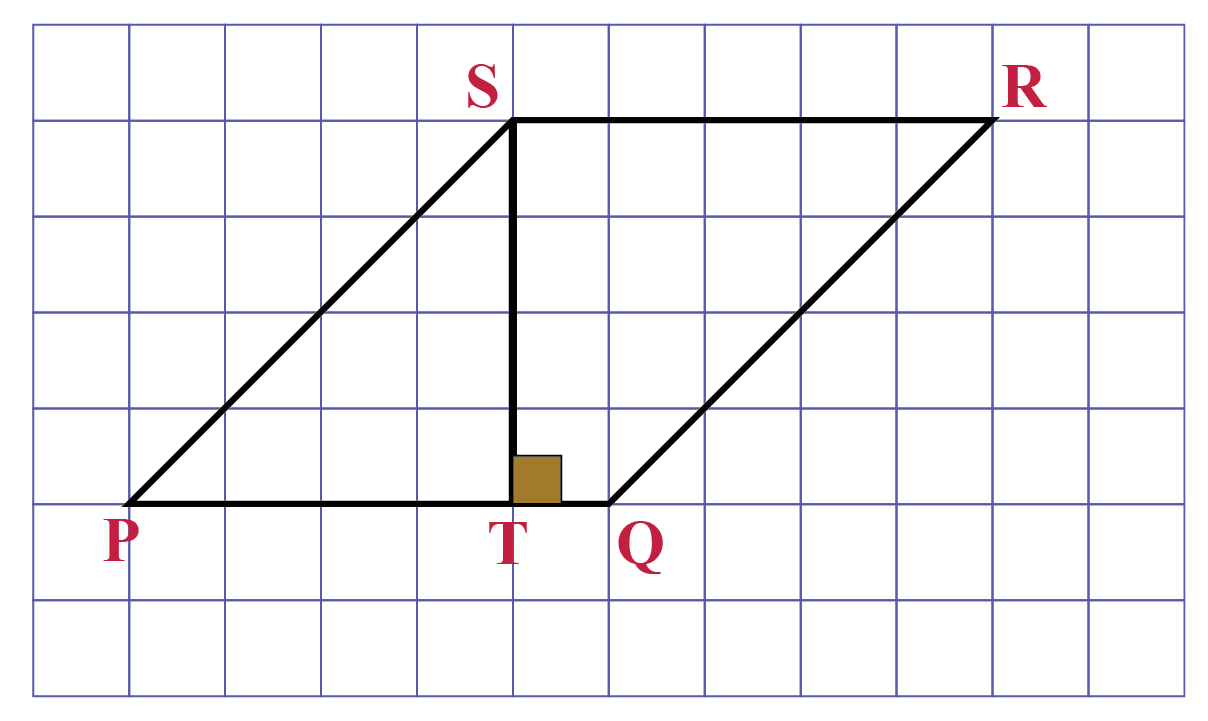From the above figure: \begin{align}\text{Total number of complete squares} &= 16\\\text {Total number of half squares} &= 8\end{align}

\begin{aligned}\text{Area} & = {16 +\frac{1}{2} \times 8} \\[0.2cm]&= 16 + 4\\[0.2cm]& = 20\ units^2\end{aligned}

In the figure, we have \begin{align}\text{ST}{\perp}\text{PQ}\end{align}

By counting the squares, we get: \begin{align}\text{Side PQ} &= 5\text{ units}\\\text {Corresponding height ST} &= 4\text{ units}\end{align}

\begin{aligned}\text{Side x height ST} & = {5 \times 4} \\[0.2cm]& = 20\ units^2\end{aligned}

The area of a parallelogram is base times altitude.Think Tank
1. Can a kite be called a parallelogram?
2. What elements of a trapezoid should be changed to make it a parallelogram?
3. Can there be a concave parallelogram?
4. Can you find the area of parallelogram without knowing its height?

## Formula of Area of a Parallelogram

### Area of Parallelogram Proof

Let's do an activity to understand the area of a parallelogram.

• STEP-I: Draw a parallelogram (PQRS) with altitude (SE) on a cardboard and cut it.
• STEP-II: Cut the triangular portion (PSE).
• STEP-II: Paste the remaining portion (EQRS) on a white chart.
• STEP-IV: Paste the triangular portion (PSE) on the white chart joining sides RQ and SP.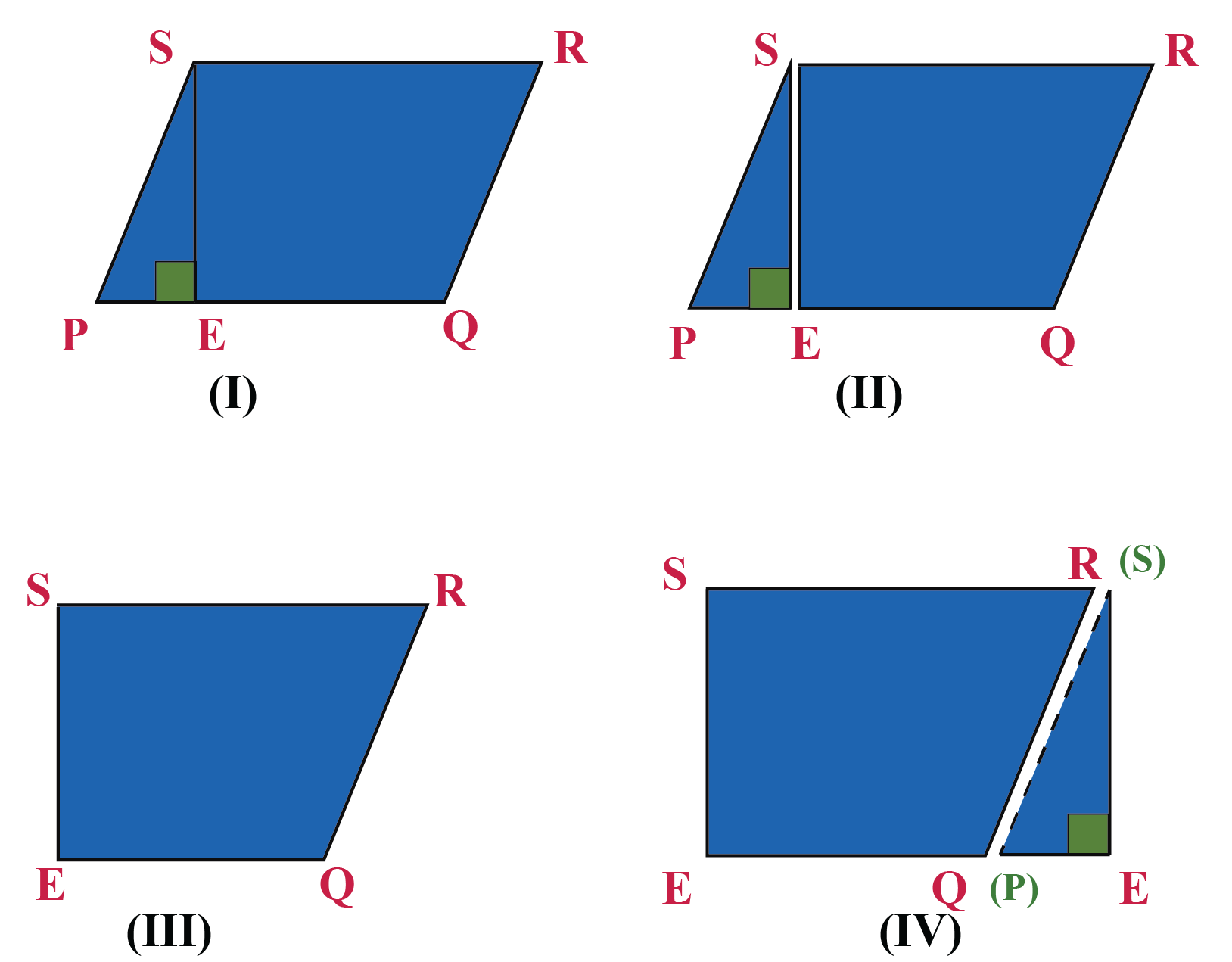Click on the show button in the simulation below, to visualize this activity.

After doing this activity, we observed that the area of a rectangle is equal to the area of a parallelogram

Note- The adjacent sides are also known as vectors. Hence, the area of parallelogram vectors is equal to the area of a parallelogram.

 \begin {align}\text {Area of Parallelogram} &\!\!=\text{Base x Height}\end{align}Challenging Questions

(A) Find the area of the following parallelograms: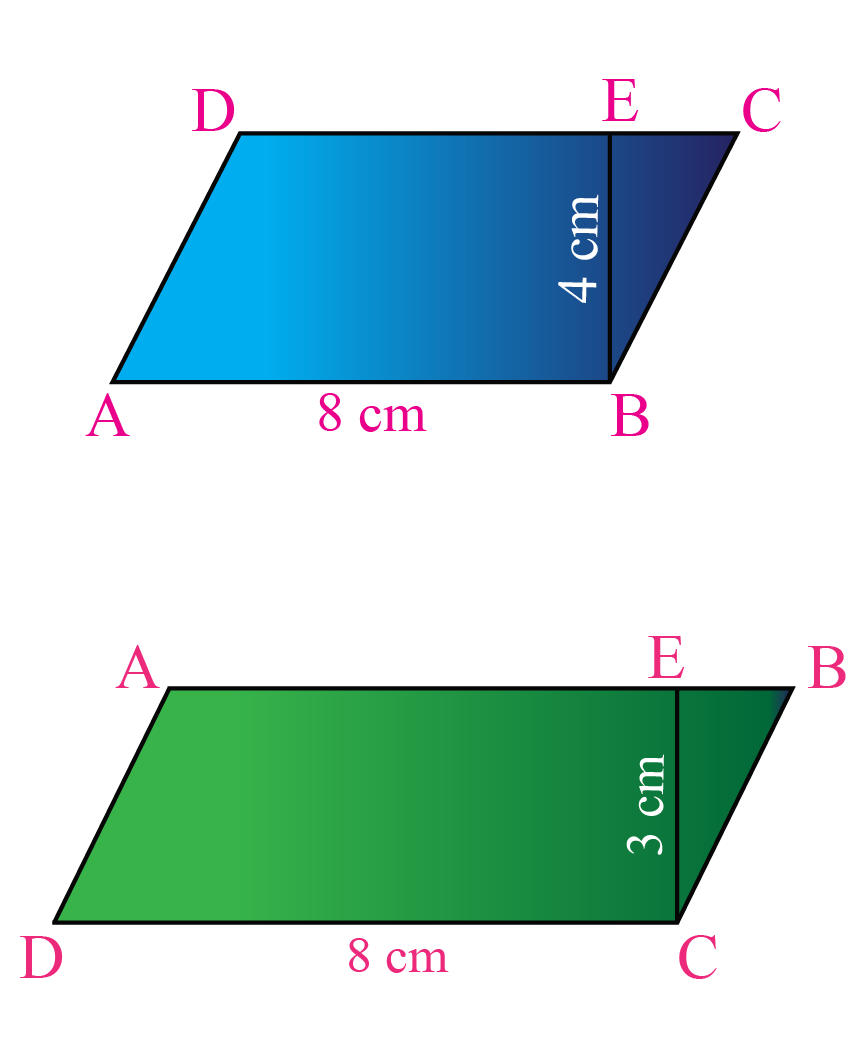(B) In a parallelogram ABCD, AB = 7.8 cm and the perpendicular from C on AB is 5 cm. Find the area.

### Area of a Parallelogram Calculator

Input the base and height in the parallelogram area calculator shown below to calculate the area of a parallelogram.

## Solved Examples

Let us look at some practical problems on how to calculate the area of a parallelogram.

 Example 1

The adjacent sides of a parallelogram are 10 cm and 6 cm.

The altitude corresponding to side 10 cm is 5 cm.

Find the area and the length of the altitude corresponding to its adjacent side.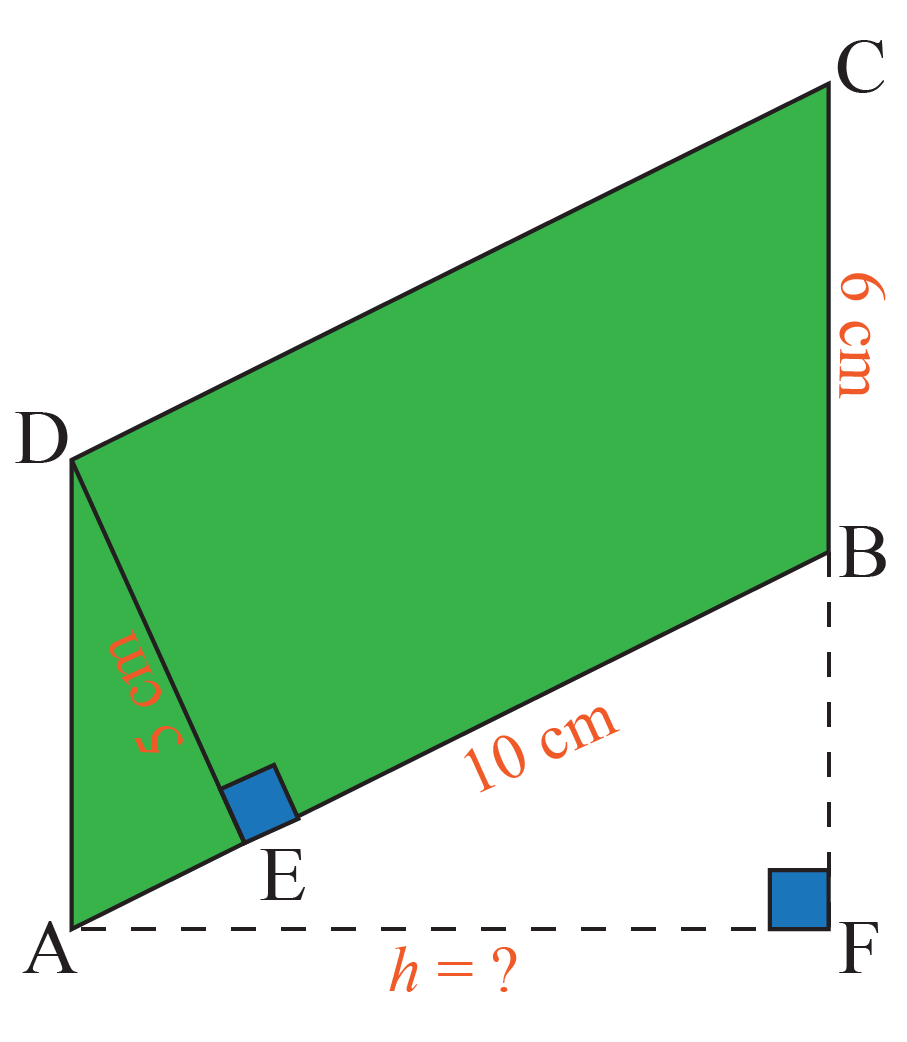Solution

Let $$ABCD$$ be a parallelogram where $$DE{\perp}AB$$, $$AF{\perp}BC$$\begin {align}\text {Area of Parallelogram ABCD} &= (10)\times (5 ) \\&= 50\ cm^2\\\text {Length of BC} &= 6\ cm\\\text {Length of Altitude AF} &= (50 \div 6) \\&= 8.3\ cm \end{align}

 $$\therefore$$ Area= 50 $$cm^2$$ Altitude= 8.3 $$cm$$
 Example 2

ABCD is a solar cell sheet in the shape of a parallelogram.

Given that, $$\ DE{\perp}AB$$, $$\ AB=20\ cm$$ and $$\ AD=10\ cm$$, $$DE=8\ cm$$.

Find the area of the solar cell sheet.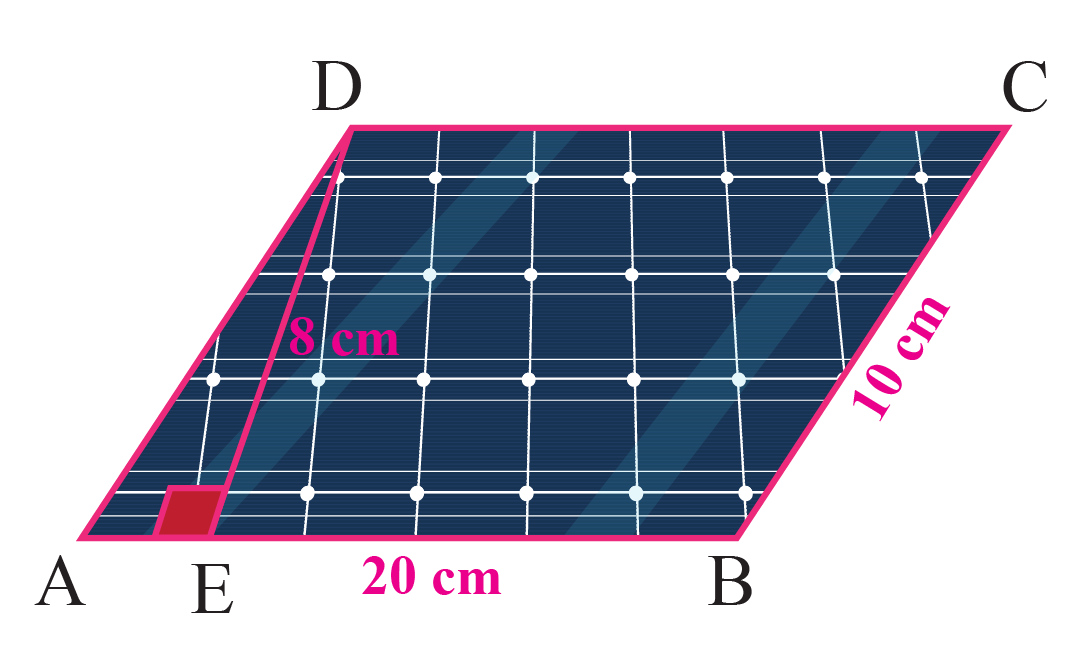Solution

\begin {align}\text{Area of the solar cell sheet} &= B\times H \\&=(20)\times(8) \\&=160\ cm^2\end{align}

 $$\therefore$$ Area of solar cell sheet =160 $$cm^2$$
 Example 3

Priya's kitchen has flowery tiles in the shape of a parallelogram.

Priya measured the dimensions of the adjacent sides of the tiles as AB = 8 cm and BC = 6 cm and altitude is 4 cm.

Can you help Priya calculate the perimeter and the area of each tile?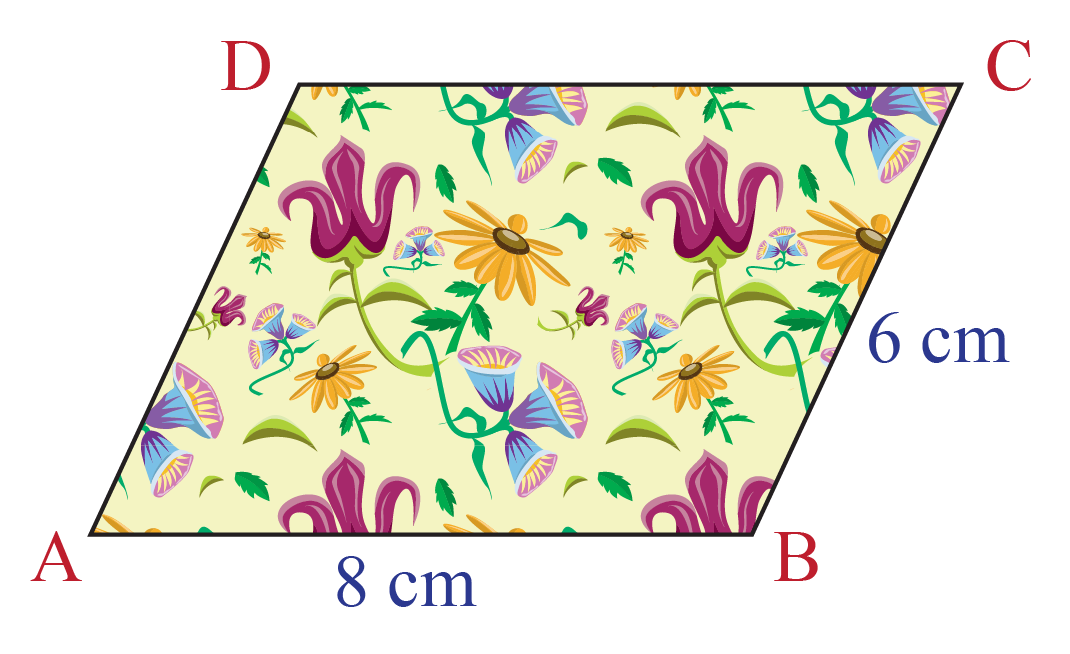Solution

Using the property of a parallelogram that states opposite side are equal, we have,

\begin{aligned}\text{Perimeter of the tile} &= {2(a + b)} \\[0.2cm]&= {2(8 + 6)} \\[0.2cm]&= 28\ cm\end{aligned}

\begin{aligned}\text{Area of the tile} &= {B \times H} \\[0.2cm]&= {8 \times 4} \\[0.2cm]&= 32 cm^2 \end{aligned}

 $$\therefore$$ Perimeter of the tile = 28 $$cm$$ Area of the tile =  32$$cm^2$$
 Example 4

The area of a playground is $$\ 2500\ cm^2$$ with one side measuring 250 cm.

Find the corresponding altitude.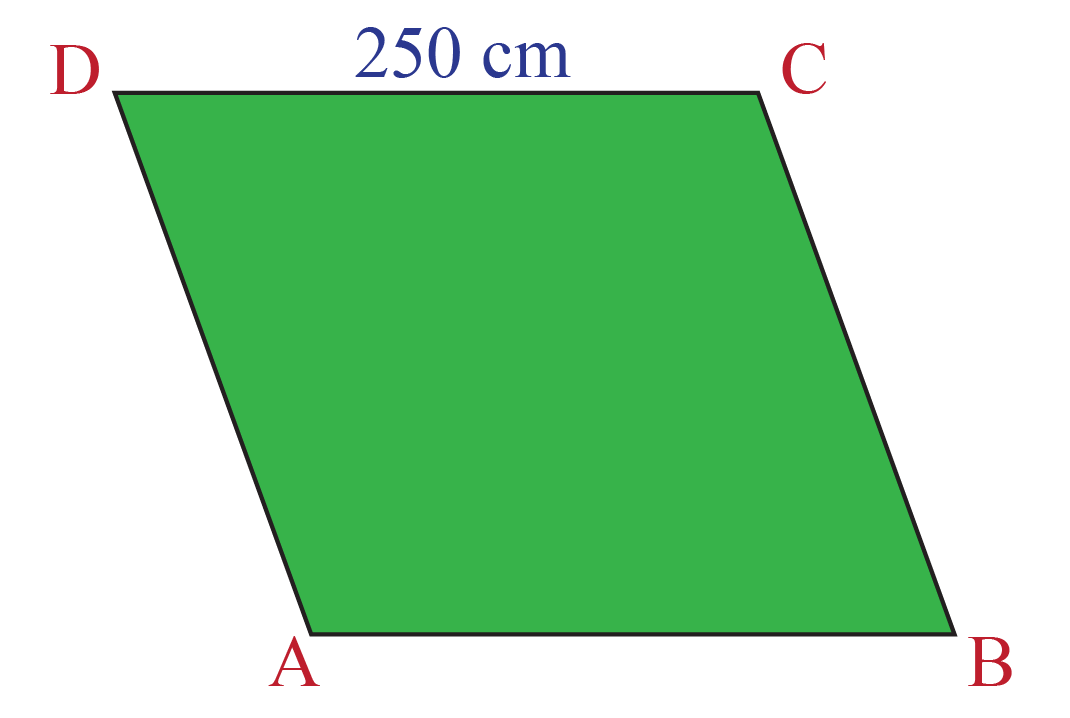Solution

\begin {align}\text {Area of the playground} &= 2500\ cm^2\\\text{Side of a playground} &= 250\ cm\\\text{corresponding altitude} &= \frac{2500}{250}\\\ &=10\ cm\end{align}

 $$\therefore$$ Corresponding altitude = 10 $$cm$$
 Example 5

Phoebe has a floral design on the floor of her bedroom.

There are 250 tiles on her floor.

Each tile is in the shape of a parallelogram of height 2 cm and base 4 cm.

Find the cost of polishing 1 tile if it costs $$\ Rs. 40$$ per square cm.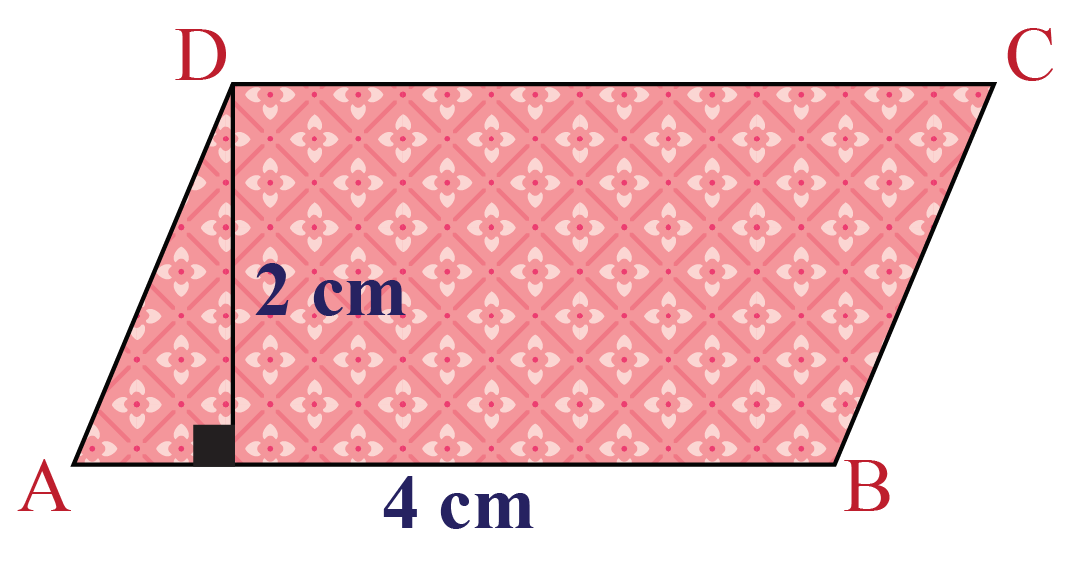Solution

\begin{aligned}\text{Base of the tile} &= {4\ cm } \end{aligned}

\begin{aligned}\text{Height of a tile} &= {2\ cm } \end{aligned}

\begin{aligned}\text{Cost of polishing 1 tile} &= {Rs. 40\times 8 } \\[0.2cm]&= Rs. 320 \end{aligned}

\begin{aligned}\text{Area of 1 tile} &= {4\times 2 } \\[0.2cm]&= 8\ cm^2 \end{aligned}

 $$\therefore$$ Cost of Polishing 1 tile = $$Rs. 320$$

## Interactive Questions

Here are a few activities for you to practice. Select/Type your answer and click the " Check Answer" button to see the result.

## Let's Summarize

We hope you enjoyed learning about the area of a parallelogram with the simulations and practice questions. Now, you will be able to easily solve problems on the area of parallelogram vectors, area of parallelogram proofs, and area of a parallelogram without height, and use the area of parallelogram calculator.

At Cuemath, our team of math experts is dedicated to making learning fun for our favorite readers, the students!

Through an interactive and engaging learning-teaching-learning approach, the teachers explore all angles of a topic.

Be it worksheets, online classes, doubt sessions, or any other form of relation, it’s the logical thinking and smart learning approach that we, at Cuemath, believe in.

## 1. How to find the height of a parallelogram?

If the area of a parallelogram is given, the formula for height is $$H=\frac{Area}{Base}$$.

## 2. How to find the area of an irregular parallelogram?

By rearranging the given irregular parallelogram into a regular figure such as a triangle, we can easily find the area of an irregular parallelogram.

## 3. The area of a parallelogram is base times height. Is this statement true?

Yes, the area of a parallelogram is A = B x H square units.

More Important Topics
Numbers
Algebra
Geometry
Measurement
Money
Data
Trigonometry
Calculus